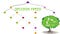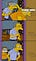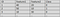# Decision Tree 101!Decision Tree

The decision tree is one of the tree-based algorithms in the machine learning domain. It is highly intuitive and easy to understand which makes it super useful in solving some of the classic machine learning problems.

So, without wasting much time we’ll dive deep into the nitty-gritty details of this algorithm.

What is a Decision Tree?

• The decision tree can be used to handle categorical and numerical data.

# K-Nearest Neighbors in a nutshellK Nearest Neighbor

You’re the average of the five people spend the most time with.

The above quote closely sums up what K-Nearest Neighbors algorithm is. The only difference is that in KNN the number of friends may vary.

K-Nearest Neighbors is a very simple but intuitive machine learning algorithm and we’ll deep dive into the nitty-gritty details of this algorithm.

What is K-Nearest Neighbors Algorithm?

K-Nearest Neighbors is a classification algorithm(can be used for regression also) that works on the basic principle that the points belonging to the same class should be closer to each other. …

# Evaluating our Machine Learning model Performance

Model Evaluation is one of the most important steps in developing a machine learning pipeline. Just imagine, designing a model and then straight away deploying it on production. And Suppose, your model is being used in the medical domain, then it may lead to the death of multiple people(if your model performs poorly). But don’t worry, this article will provide you with all the tools needed to evaluate and improve your model performance.

Classification Problems

As we know, classification problems are those problems in which the output is a discrete value.For e.g. spam detection,cancer detection etc.

For the scope of…

# Why Activation Functions?

In this article we’ll address the following topics:

• Why we need activation functions
• Different kind of activation functions
• Advantages and disadvantages of different activation functions

Why do we need Activation Functions?

Let’s take an example of a single neuron. Each neuron performs two functions:

• Calculate the weighted sum of all input features
• Pass this into an activation function.
`z = w1*x1 + w2*x2 + w3*x3 - (Eq.1)(where x1,x2,x3 are input features and w1,w2,w3 are weights)a = f(z) where f is the activation function.`

So the activation function basically provides a non-linearity to z, which helps in learning complex functions…

# Preparing for Optimization Algorithms for data science interviews

One of the most basic data science interview questions we are asked is about different optimization algorithms in deep learning. This article will be a one stop draft for all the information regarding optimization algorithms.

What we’ll cover?

We’ll cover the following optimization algorithms with their pros and cons:

1. Algorithms based on weight update rule.
• Gradient Descent
• Momentum Based Gradient Descent
• Nesterov Accelerated Gradient Descent

2. Batch size based optimization algorithms.

• Batch Gradient Descent
• Mini Batch Gradient Descent
• Stochastic Gradient Descent

3. Adaptive learning rate based optimization algorithms.

• Adagrad
• RMSProp
• Adam

So, without wasting much time we’ll start with our…

# Bias and Variance in the Deep Learning era

One of the concepts of machine learning that is easy to learn but difficult to master is Bias and Variance. It’s like Artificial Intelligence for newbies, they talk a lot about it but don't’ know how it works internally(no offense, guys).

Understanding Bias and Variance

Whenever we are training our model, what we want is that the model have a minimum loss and we get a decision boundary that differentiates classes with high accuracy. But that is not always what we get.

We take a dataset and divide it into 3 parts: train set, validation set, and test set. The…

# A quick guide to getting started with NumPy

Whenever someone decides to enter into the world of data science, the one library he needs to learn is NumPy. NumPy Stands for Numerical Python and this article will be like a crash course in NumPy.

So Why Numpy?

NumPy is similar to python list and can perform most of the operations python lists perform but NumPy has its own advantages for which it is the preferred library for handling large numerical data. Some of these are:

• NumPy, unlike python lists, has homogenous data type because of which it can be densely packed into memory and it also frees memory…

# Deep Dive into Logistic Regression

Readers Warning: Hold your seat guys, since this is going to be a long and comprehensive article covering all the nitty-gritty details of Logistic Regression.

We’ll first discussion about all the ingredients required and then see how these ingredients come together to prepare an awesome algorithm.

So, without wasting any time let’s start with our article.

When to use Logistic Regression?

You all must have heard of classic machine learning problems like cats v/s dogs, bening v/s malignant tumor, or basically, we can say, where ever we need to classify between two classes i.e. …

# Neural Networks and the Power of Universal Approximation Theorem.

We all have heard about neural networks and how it is able to learn any complex function. But let me tell you it’s no real magic rather a combination of some cool algorithms and theorems put together such that they manage to replicate human level accuracy.

## Problem Statement

Let’s take a problem statement for binary classification. Suppose you are given a dataset in which you are given some features and based on those features you have to tell whether a person has cancer (class 1) or not (class 0).Binary Classification Dataset

Task

Now our task is to find a function which can map our…

# The Recursion Trap.

I always used to think why in the world do we have recursion(bcoz I never actually got a hang of it) and it used to be the case with almost all my friends because recursion really is like a trap unless you get a broader look at its beauty. I finally realized this few days back while flipping my textbook pages and it finally struck me, the Eureka moment!!! just kidding, I watched a video and the guy explained it really well.

To understand recursion, you need to understand recursion.

That’s true, first understand what recursion is, not as a…## Arpan Srivastava

AI enthusiast with a desire to share ideas